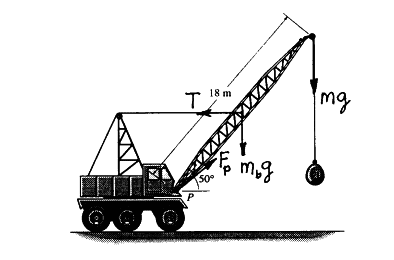# How to Take the sum of moments

How to Take the sum of moments !!!

## Homework Statement

Hi i am doing static equilibrium exercises in textbook.

## Homework Equations

But I dont know how to find sum of moments at P when its come to angle.
This is a drawing## The Attempt at a Solution

I have No idea !!! I was trying to get my head around But still cant Do it !!

SteamKing
Staff Emeritus
Homework Helper
Well, the diagram attached to the OP doesn't contain enough information anyway. Is there problem text which accompanies the diagram which you haven't supplied? Or are you to assume that the weight of the boom is located at half its length from point P?

NascentOxygen
Staff Emeritus
A couple of reasonable assumptions seem in order here.

To find the moment that a force contributes, you multiply the force by the perpendicular distance to the line of action of that force. So you have the opportunity to demonstrate your skill with trigonometry here.Last edited:
CWatters
Homework Helper
Gold Member
What Nascent said.

Making reasonable assumptions it seems possible to calculate T. For example T appears to be connected roughly half way up the boom. There is enough info to calculate the perpendicular distances that Nascent refers to. I would assume the boom is very light weight compared to the wrecking ball.

Yes, the centre of mass at mid weight and pivot at p.

CWatters